# Understanding power factor and the need for power factor correction

Author:
Arun Ananthampalayam, CUI

Date
09/24/2013PDF
##### Due to international legislation governing power factor and harmonic distortion, PFC is now a serious industry concern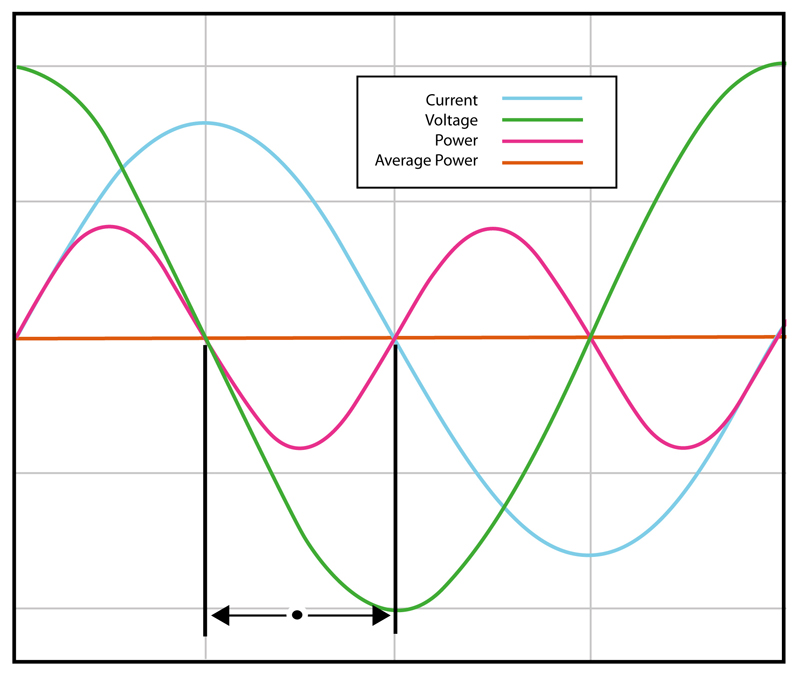Click image to enlarge

Figure 1: An ideal waveform with a power factor of 0

Until the era of switching power supplies, Power Factor - and power factor correction - wasn't a big concern for all but a handful of electrical engineers working with large electric motors, and other, generally high-power and industrial, electric loads. Now, thanks to international legislation governing power factor and harmonic distortion, this subject is high on the list of concerns for many engineers designing systems for global use. A Quick Introduction to Power Factor Power factor (pf) is the ratio between real power (P) flowing to the load, and the apparent power in the circuit (S): pf = P/S. It is a sinusoidal waveform and therefore expressed as a dimensionless number between -1 and 1. Real power is measured in watts (W) and apparent power in volt-amps (VA). For a purely resistive load, the two figures are identical; for a reactive load the arithmetic for the apparent power produces the same figure, that is, the product of the RMS values of voltage and current. However, to find the actual (real) power delivered to the load, the instantaneous product of voltage and current must be integrated over the complete sine-wave cycle (see Figure 1).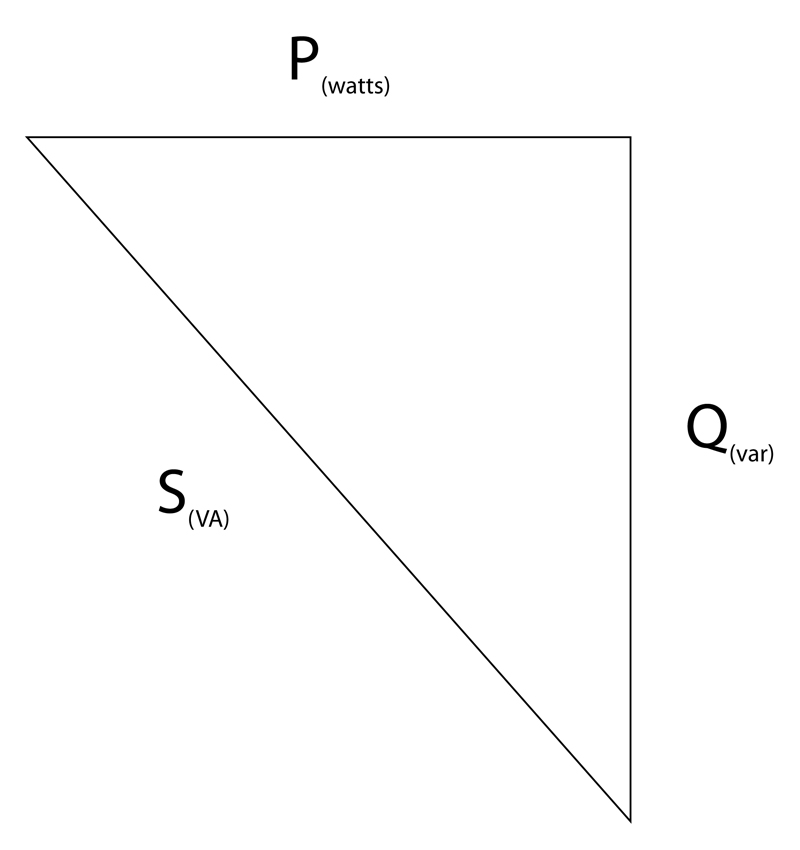When current is leading or lagging voltage, the value of that integral will always be less than the value for the in-phase case over the same interval. This reflects the attribute of an inductor or a capacitor to act as an energy store; at various points through the AC cycle the reactive component is either storing energy, or returning it to the system. The apparent power is the vector sum of the true power and the reactive power (Q), measured in reactive volt amperes (var); conventionally, this relationship is expressed as: P = S cos? or P2+Q2=S2 The relationship is conventionally visualized in a right-angled triangle vector diagram (see Figure 2). This is a basic definition and works for pure sinusoids; non-sinusoidal waveforms are more complex, but can be represented by a series of harmonic sinusoids and therefore the same basic principles apply.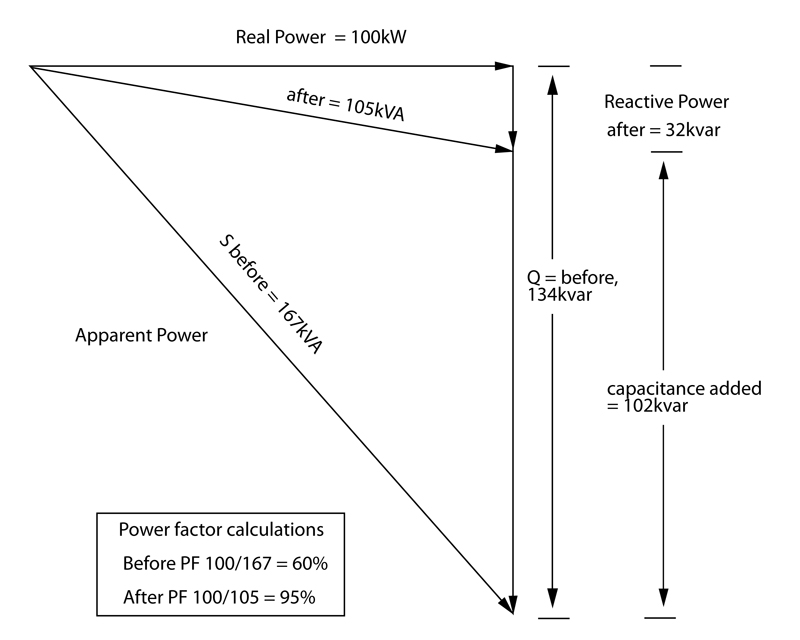Power Factor Correction The solution to excess harmonics is to use power factor correction (PFC). This shapes the input current of the power supply to maximize the real power level from the mains and minimize harmonic distortion (see Figure 4). Ideally, the electrical appliance should present a load that resembles a linear load, such as a simple resistor, rather than the reactive load of an uncorrected switching power supply. This corrected waveform minimizes losses as well as interference with other devices being powered from the same source. Compensation for low power factor can be by passive or active devices. The simplest case is highlighted in electric motor applications. Naturally, as wound machines, they provide a highly inductive load, and adding capacitors to the supply network has long been standard practice. However, even this case may not be entirely simple. For example, the designer of such a network has to take care not to create unwanted resonant effects. Variable power factor in the load may be accommodated by an adaptive scheme to connect reactive elements as required and in high-power contexts (MW scale) rotating-machinery solutions can be applied.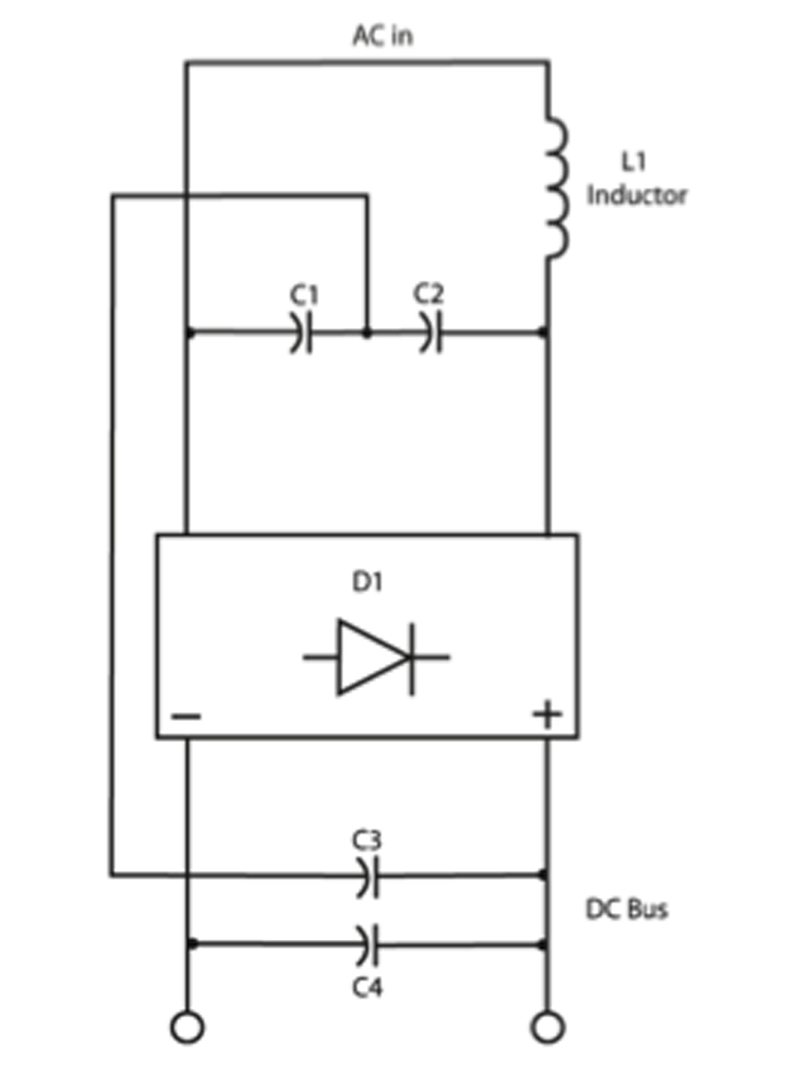Passive power factor correction in the form of filtering can be effective, within limits, and has the effect of reducing the higher-order current harmonics that, as noted above, contribute to degraded power factor. Such techniques involve putting a low-pass filter in the input side of the power supply to suppress higher-order harmonic components, and then compensating lead/lag characteristics as with conventional power factor. The downside to a passive PFC design is that large (both by value, and physically) inductors and/or capacitors may be required. Additionally, there are limitations to the input range and power rating when implementing this scheme (see Figure 5). Passive PFC circuits are generally able to achieve a power factor in the range of 0.70-0.75. The existence of, and rapid progress in, high-speed, high-current capacity semiconductor switches now make available the option to achieve a power factor up to 0.99. Active power factor correction is the scheme that is most widely applied in present-day designs. A switching pre-regulator stage is placed in the input current path of the supply. That regulator is designed not only to maintain a constant DC voltage to feed the main converter stage of the power supply, but also to draw current from the input in-phase with the incoming AC voltage waveform. And while adding a switching stage does impose some extra losses, and some extra cost, there are compensating savings in the form of smaller passive filtering components, and in the supply's main converter. Summary Power factor is on the list of concerns for designers of virtually every device that draws significant power from a mains socket, as well as for engineers in heavy-electrical sectors. The power factor target, based on legislation, plus efficiency, component cost, and volume/board space, needs to be considered. CUI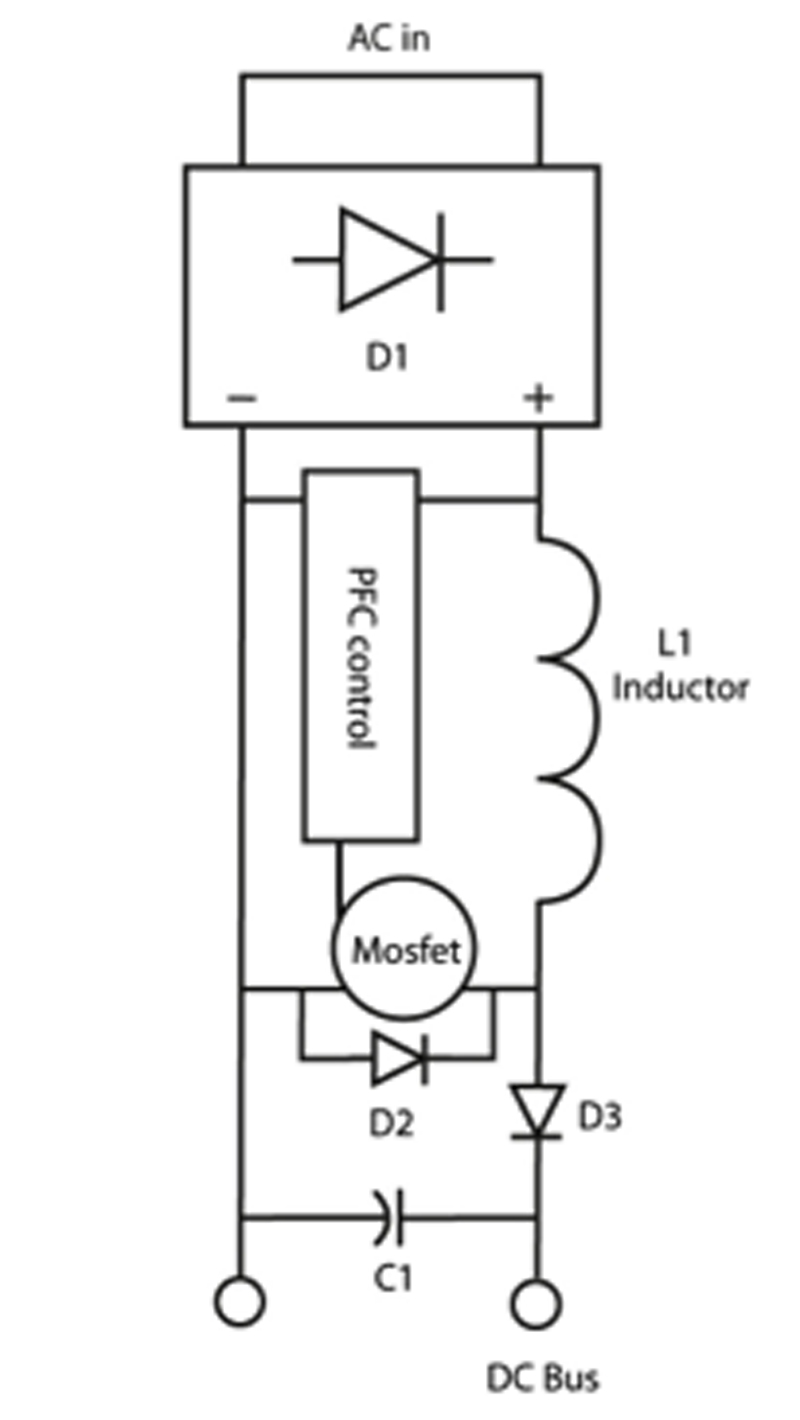### RELATED#### 20Gbps Multi-Channel Mux/Demux For Video Signal Routing

May 5,2021May 4,2021#### Thermoelectric Coolers Increase PCR Cycling Reliability

May 3,2021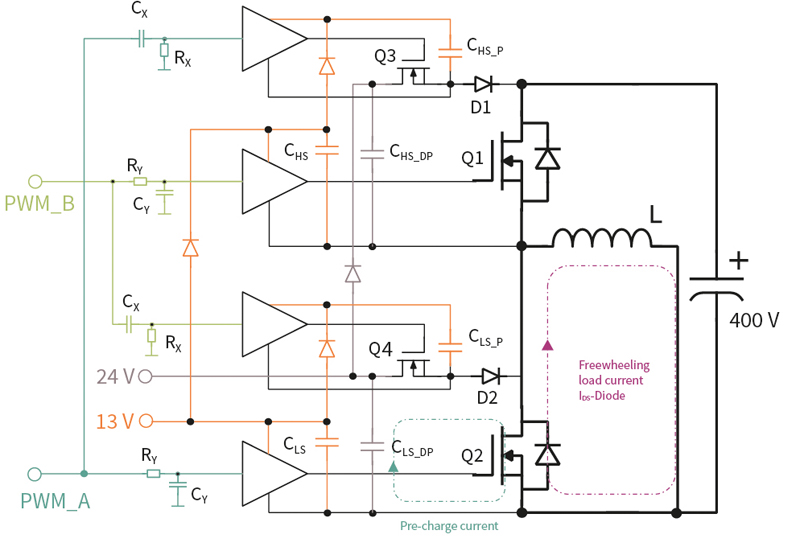May 3,2021